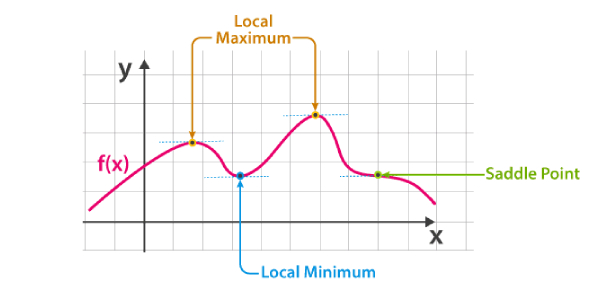# Application Of Derivatives: Math Trivia Quiz

15 Questions | Attempts: 1180
ShareSettingsDo you know the applications of derivatives? Many people have a negative feeling about math and think they won’t understand how to solve fundamental problems. Problems involving derivatives are easy to solve, as all one has to follow specific procedures. The quiz below is a perfect way to test out your solving skills. Do give it a shot as you refer to your notes and keep a lookout for more quizzes like it!

• 1.
Find the interval in which the function f given by f(x) = log sin x is strictly increasing on and strictly decreasing on.
• A.
• B.
• C.

Both A & B

• D.

None of these

• 2.
Find the rate of change of the area of a circle with respect to its radius r when r = 4 cm
• A.

• B.

• C.

• D.

• 3.
Find the points on the curve x2 + y2- 2x-3=0 at which the tangents are parallel to the x-axis.
• A.

(1, 2) and (1, −2)

• B.

(1, -2) and (1, 2)

• C.

(-1, 2) and (1, −2)

• D.

(-1, 2) and (1, 2)

• 4.
It is given that at x = 1, the function x4− 62x2 + ax + 9 attains its maximum value, on the interval [0, 2]. Find the value of a.
• A.

120

• B.

110

• C.

-110

• D.

-120

• 5.
For the curve y = 4x3 − 2x5, find all the points at which the tangents passes through the origin.
• A.

(0, 0), (1, 2), and (1, −2)

• B.

(0, 0), (1, 2), and (−1, −2)

• C.

(0, 0), (1, 2), and (−1, 2)

• D.

(0, 0), (1, -2), and (−1, −2)

• 6.
Sand is pouring from a pipe at the rate of 12 cm3 /s. The falling sand forms a cone on the ground in such a way that the height of the cone is always one-sixth of the radius of the base. How fast is the height of the sand cone increasing when the height is 4cm?
• A.
• B.
• C.
• D.
• 7.
Find the points on the curve y = x3 at which the slope of the tangent is equal to the y-coordinate of the point.
• A.

(0, 0) and (2, 27)

• B.

(0, 0) and (3, 27)

• C.

(0, 0) and (3, 25)

• D.

(0, 0) and (3, 25)

• 8.
The length x of a rectangle is decreasing at the rate of 5 cm/minute and the width y is increasing at the rate of 4 cm/minute. When x = 8 cm and y = 6 cm, which of the following is true?
• A.

Perimeter is decreasing at the rate of 2 cm/min

• B.

Area of the rectangle is increasing at the rate of 2 cm2/min

• C.

Both A & B

• D.

None of these

• 9.
The volume of a cube is increasing at the rate of 8 cm3 /s. How fast is the surface area increasing when the length of an edge is 12 cm?
• A.
• B.
• C.
• D.
• 10.
Find both the maximum value and the minimum value of 3x4 − 8x3 + 12x2 − 48x + 25 on the interval [0, 3]
• A.

The absolute maximum value is 25

• B.

The absolute minimum value is − 39

• C.

Both A & B

• D.

None of these

• 11.
Find the equations of the tangent and normal to the hyperbola  at the point  .
• A.
• B.
• C.
• D.
• 12.
Find the equation of the tangent to the curve  which is parallel to the line 4x − 2y + 5 = 0.
• A.

48x + 24y = 23

• B.

48x – 24y = 25

• C.

48x – 24y = 23

• D.

48x – 24y = 28

• 13.
A wire of length 28 m is to be cut into two pieces. One of the pieces is to be made into a square and the other into a circle. What should be the length of the two pieces so that the combined area of the square and the circle is minimum?
• A.
• B.
• C.
• D.
• 14.
Find two positive numbers and such that their sum is 35 and the product x2y5 is a maximum
• A.

10 & 25

• B.

15 & 20

• C.

5 & 30

• D.

None of these

• 15.
Find the semi-vertical angle of the cone of the maximum volume and is of given slant height.
• A.
• B.
• C.
• D.

## Related TopicsBack to top
×

Wait!
Here's an interesting quiz for you.